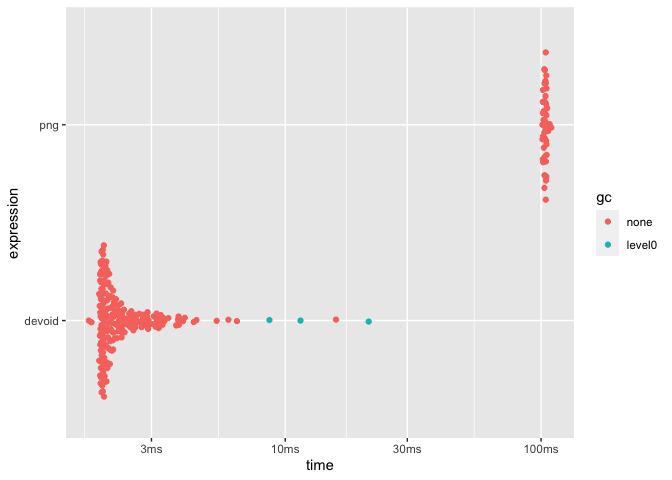# devoid

This package provides a graphic device that does no operations. This makes it suitable for benchmarking functions that produce graphics as it removes the device implementation from the equation. In contrast to the `nulldev()` function from R.devices package this device is a true device implementation that simply does nothing rather than calling `pdf()` with a temporary file connection.

## Installation

You can install `devoid` with the remotes package:

``````# install.packages('remotes')
remotes::install_github('r-lib/devoid')``````

## Example

`devoid` provides a single function `void_dev()` which is used much like any other device:

``````library(devoid)

void_dev()
plot(1:10, 1:10)
dev.off()
#> quartz_off_screen
#>                 2``````

Using it we can now see how much drawing time e.g. the png device is responsible for:

``````point_coord <- seq_len(1e4)
void_plot <- function() {
void_dev()
plot(point_coord, point_coord)
dev.off()
NULL
}
png_plot <- function() {
png(tempfile())
plot(point_coord, point_coord)
dev.off()
NULL
}

res <- bench::mark(devoid = void_plot(), png = png_plot(), min_iterations = 50)
plot(res)